CSE 5311 Section 501 Fall 1998
Homework 3
Due: February 25, 1998, in class

1. Print out the table m created by performing the memoized version of matrix-chain on the sequence of dimensions 30, 35, 15, 5, 10, 20, 25.

```m is
0 15750  7875  9375 11875 15125
0     0  2625  4375  7125 10500
0     0     0   750  2500  5375
0     0     0     0  1000  3500
0     0     0     0     0  5000
0     0     0     0     0     0```
2. Use the algorithm given in class to determine an LCS of (1,0,0,1,0,1,1,1) and (0,1,0,1,1,0,1,1,0).

Here is the table computed by LCS-LENGTH, where ``UL'' represents an arrow pointing up and left, ``UU'' represents an arrow an up arrow, and ``LL'' represents a left arrow.

```(0 --) (0 --) (0 --) (0 --) (0 --) (0 --) (0 --) (0 --) (0 --) (0 --)
(0 --) (0 UU) (1 UL) (1 LL) (1 UL) (1 UL) (1 LL) (1 UL) (1 UL) (1 LL)
(0 --) (1 UL) (1 UU) (2 UL) (2 LL) (2 LL) (2 UL) (2 LL) (2 LL) (2 UL)
(0 --) (1 UL) (1 UU) (2 UL) (2 UU) (2 UU) (3 UL) (3 LL) (3 LL) (3 UL)
(0 --) (1 UU) (2 UL) (2 UU) (3 UL) (3 UL) (3 UU) (4 UL) (4 UL) (4 LL)
(0 --) (1 UL) (2 UU) (3 UL) (3 UU) (3 UU) (4 UL) (4 UU) (4 UU) (5 UL)
(0 --) (1 UU) (2 UL) (3 UU) (4 UL) (4 UL) (4 UU) (5 UL) (5 UL) (5 UU)
(0 --) (1 UU) (2 UL) (3 UU) (4 UL) (5 UL) (5 LL) (5 UL) (6 UL) (6 LL)
(0 --) (1 UU) (2 UL) (3 UU) (4 UL) (5 UL) (5 UU) (6 UL) (6 UL) (6 UU)```

The longest common subsequence is (1, 0, 1, 0, 1, 1).

3. Can the black-heights of nodes in a red-black tree be maintained as fields in the nodes of the tree without affecting the asymptotic performance of any of the red-black tree operations? Show how, or argue why not (this is Exercise 15.2-3).

Yes, by Theorem 15.1, because the black-height of a node can be computed from the information at the node and its two children. Actually, the black-height can be computed from just one child's information: the black-height of a node is the black-height of a red child, or the black height of a black child plus one.

4. Give a dynamic-programming solution to the 0-1 knapsack problem that runs in O(nW) time, where n is the number of items and W is the maximum weight of items that the thief can put in his knapsack (this is Exercise 17.2-2).

The textbook provides an optimal substructure observation: let i be the highest-numbered item in an optimal solution S for W pounds and items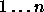. Then S' = S - {i} is an optimal solution for W -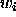pounds and items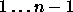, and the value of the solution S is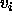plus the value of the subproblem solution S'.

Define c[i,w] to be the value of the solution for items 1..i and maximum weight w. Then

```            +---
| 0                                if i=0 or w=0
c[i,w] = | c[i-1,w]                         if w[i] > w
| max(vi + c[i-1,w-wi], c[i-1,w]   if i>0 and w>=w[i]
+---```

This says that the value of a solution for i items either includes item i, in which case it isplus a subproblem solution for i-1 items and the weight excluding, or does not include item i, in which case it is a subproblem solution for i-1 items and the same weight. The better of these two choices should be made.

The algorithm takes as input the maximum weight W, the number of items n, and the two sequences v = (v1, v2, ..., vn) and w = (w1, w2, ..., wn). It stores the c[i,j] values in a table c[0..n, 0..W] whose entries are computed in row-major order. At the end of the computation, c[n,W] contains the maximum value the thief can take.

```   Dynamic-0-1-Knapsack(v, w, n, W)
for w = 0 to W
c[0,w] = 0
for i = 1 to n
c[i,0] = 0
for w = 1 to W
if wi <= w
then if vi + c[i-1,w-wi] > c[i-1,w]
then c[i,w] = vi + c[i-1,w-wi]
else c[i,w] = c[i-1,w]
else c[i,w] = c[i-1,w]```

The set of items to take can be deduced from the c table by starting at c[n,W] and tracing where the optimal values came from. If c[i,w] = c[i-1,w], item i is not part of the solution, and we continue tracing with c[i-1,w]. Otherwise, item i is part of the solution, and we continue tracing with c[i-1, w-wi]. This algorithm requires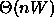steps,time to fill in the c table ((n+1)*(W+1)) entries each requiring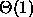time to compute), and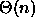time to trace the solution since it starts in row n of the table and moves up 1 row at each step.

5. Generate a dynamic programming solution to the calculation of World Series odds. Compare the run time of your approach with therun time of the straightforward approach. In the World Series, two teams (A and B) are playing a match to see who is the first to win n games. Each team has a 50% change of winning any game. The problems is to compute P(i,j), the probability that if A needs i games to win and B needs j games to win, that A will eventually win the match. For example, in the World Series, if the Dodgers have won two games and the Yankees have won one game, then i=2, j=3, and P(2,3) is 11/16.

Note that if i=0 and j>0, then team A has already won and P(0,j) = 1. Similarly, P(i,0) = 0 for i>0. If i and j are both greater than 0, then P(i,j) must be the average of P(i-1,j) (the probability that A wins the match if it wins the next game) and P(i,j-1) (the probability that A wins the match if it loses the next game).

A dynamic programming solution fills in P[i,j] entries in diagonals beginning at i=0,j=0 and proceeding up and to the left along diagonals representing entries with a constant value of i+j.

```   Odds(i, j)                                             TIMES
for s = 1 to i+j                                     n+1
P[0,s] = 1.0                                       n
P[s,0] = 0.0                                       n
for k = 1 to s-1                                  n*s
P[k,s-k] = (P[k-1,s-k] + P[k,s-k-1]) / 2      n(s-1)
return(P[i,j])                                        1```

Assuming that n = i+j, the run time of Odds is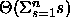or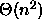, which is much more efficient than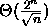.

6. Consider the problem of constructing a minimum height binary search tree for an ordering sequence of keys (for example, 1, 2, 3, 4, 5) by choosing one key to be the root (for example, 3) and then recursively constructing trees for the keys to the left (1, 2 in this case) and right (4, 5 in this case) of the root. Show that this problem exhibits optimal substructure.

In addition, consider a greedy solution that always choose the middle key of the sequence to be the root of the tree. Show that this greedy choice always appears in an optimal solution to the tree problem, although there may exist additional optimal solutions not containing the greedy choice.

To show that the problem exhibits optimal substructure, let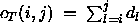be the cost of a binary search tree T built for the keys i through j. Assume we have an optimal tree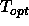for the keys i through j consisting of a root key k with left subtree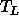containing keys i to k-1 and right subtree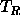containing keys k+1 to j. We can express the cost of the treeas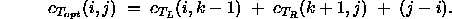The last term derives from the fact that attaching the subtreesandto the root key k adds one to the depth of each key in the subtrees. Since there are (j - i) keys in the subtrees, the last term adds one to the depth of each of these keys.

To prove optimal substructure, we need to show that the subtreesandcontained inare optimal binary search trees for the keys i to k-1 and k+1 to j, respectively. Using proof by contradiction, assume that there is a tree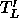having a lower cost than; or that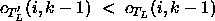. Then we can build a new treeby replacingby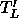in. The cost of treewill be the same as in the equation above withreplaced by. Since,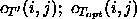. However, this contradicts the original assumption thatis the optimal tree. Therefore, the subtrees must be optimal as well, and the problem exhibits optimal substructure.

To prove greedy choice, let n = j - i + 1 be the number of keys to be stored in the BST. Minimizing the height of the BST will minimize the sum of the depths of the keys in the tree. For a binary tree with n keys, the minimum height (height of the optimal tree) is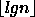. An optimal slipt of n keys into a root key and a left and a right subtree puts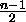keys in each subtree. Thus, the optimal key to split on is key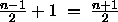. If i=1 and j=n, then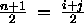. When i+j is odd, then either key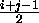or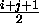will work, so we arbitrarily use the floor \(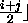. Any other choice will put an excessive number of keys in one subtree with greater depth; therefore, increasing the cost of the entire tree. Thus, choosing the middle key of the sequence as the root will be in an optimal solution.

Next, we show that the greedy choice can be made first. In particular, we show that we can choose the root node before any other node. The proof is trivial, because no matter what order we assign keys to their positions, the depths will not change and the cost will remain optimal. Therefore, since we can assign any key first, we can assign the greedy choice key first.

The above proof shows that we can make the greedy choice for the root of the entire tree. The next step is to prove by induction that we can make greedy choices at any point in the tree. Since this is equivalent to proving the problem exhibits optimal substructure, which was already done in part 1a, then we have completed the proof that the greedy choice satisfies the greedy choice property.